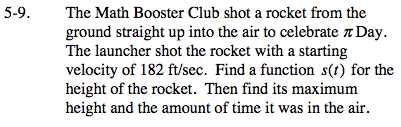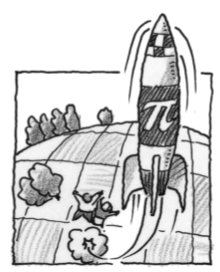### Home > CALC > Chapter 5 > Lesson 5.1.1 > Problem5-9

5-9.Start with the basic equation: s(t) = −16t² + v0t + s0 where v0 is the initial velocity and s0 is the initial height of the rocket from the ground.

The initial height of the rocket is 0 because it is shot from the ground.The initial velocity is 182 ft/sec. Thus: s(t) = −16t2 + 182t

To find the maximum height, take the derivative of the function and set it equal to zero because the maximum height occurs when the velocity is zero.

0 = −32t +182